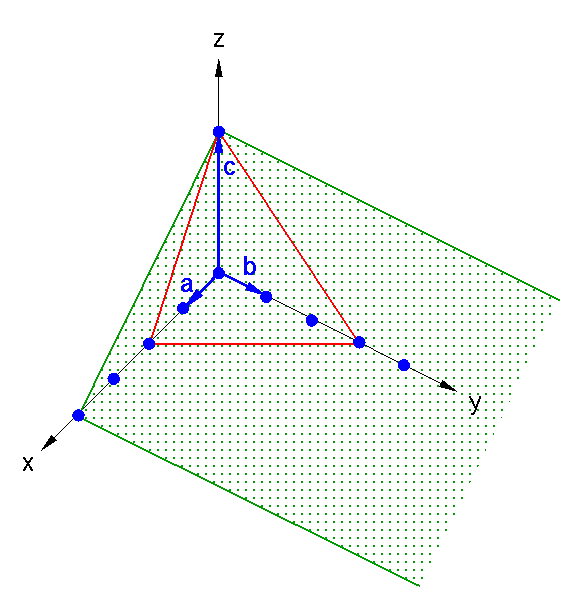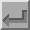Miller Planes

In order to denote any plane through a crystal lattice we use a system devised by Miller in 1839, originally to describe the crystal faces. He defined three indices h, k, l as the reciprocal cut-offs with the three crystallographic axes. The triplet is placed in brackets, (hkl), and describes a set of parallel planes with a certain relative orientation to the crystal lattice.

The following is an easy way to find the values of h, k and l.

1. Take the cut-offs of the axes x, y and z as multiples of a, b and c. (If the plane does not intersect an axis this is infinity.)
2. Take the reciprocal of each number. (The reciprocal of infinity is zero.)
3. Multiply each with the lowest common denominator to get integers.Example 1 (the red plane in the above illustration):

 1 Cut-offs: 2 3 1 2 Reciprocal: 1/2 1/3 1/1 3 Multiply by 6: 6/2 6/3 6/1 → (3,2,6)

Example 2 (the green plane):

 1 Cut-offs: 4 ∞ 1 2 Reciprocal: 1/4 1/∞ 1/1 3 Multiply by 4: 4/4 0 4/1 → (1,0,4)

This procedure gives the Miller indices (hkl) or (h,k,l) of a plane in the crystal. However, these may or may not be the same as the reflection indices, also denoted by (hkl), which define the reciprocal lattice coordinates of a diffraction spot (more about this in a later section).

The difference between the Miller indices and the reflection indices is that the Miller indices never have a common factor; this is guaranteed by the above procedure for obtaining them. However the corresponding reflection indices may or may not have a common factor, because in principle a given crystal plane gives rise to an infinite number of orders of diffraction. So, for example, the reflections with indices (-3,0,-12), (-2,0,-8), (-1,0,-4), (1,0,4), (2,0,8), (3,0,12), etc. are all X-ray reflections from the crystal planes with Miller indices (1,0,4). In fact the reflection indices differ from the Miller indices by a factor equal to the order of diffraction n. Note that in practice crystallographers are only concerned with reflection indices.Back